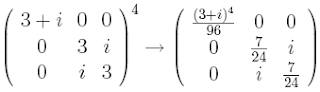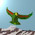## Friday, May 7, 2010

### M Theory Lesson 325

Recall that the $2 \times 2$ matrix $R_{2}^{8}$ is the identity. This has a few fun consequences for the $R_2$ factors in mixing matrices. Physicists like to think of the CKM matrix as a product of up and down factors. The properties of $R_2$ mean that the mixing factor with parameter $r$ may be itself factored into several copies of the same $R_2$ type. That is, $R(r) = R(1)R(1)R(1/r)$. At an intermediate stage, we find for example that $R(24) = R(1)R(25/23)$. Taking powers of scaled $R_2$ factors we find occasional nice results, such aswhich displays the Pythagorean triple $(7,24,25)$. There are many ways to factor these scaled matrices into two or more components.

1.Taking $R(r)^{2}$ for even $r$ generates the Babylonian primitive Pythagorean triples (in the norm square matrix). These have two numbers differing by $2$. For example, $R(24)^2$ gives $(24^2 \pm 1,48)$. Or, $R(12)^2$ gives $(24,143,145)$. Similarly, the odd numbers give triples with two numbers differing by $1$. Eg: $R(5)^2$ gives $(5,12,13)$. This is a simple consequence of the squaring, which takes $r$ in $R(r)$ to $r^2 - 1$ divided by $2r$.
2.... so $R(7)^2$ gives essentially the same factor (the inversion) as $R(3)^4$, and there are countless other such relations.